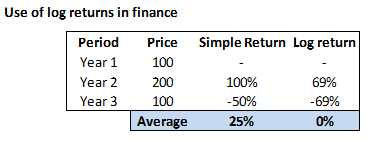# Why stock returns are calculated in Log scale?

6 Likes

Very interesting question Sam. Not many traders actually know this...

Consider this ... you invested Rs.100 in a stock in year 1.... it grows to Rs.200 in year 2 giving you a return of 100%. In year 3 the stock price comes back to Rs.100 from Rs.200 giving you a negative return of 50%. What do you think is the average return?

To calculate the average you are most likely going to do this... [+100 + (-50)] / 3 = 25%

So the arithmetic average is suggesting that you have earned an average of 25%. But in reality you have earned 0%.

Do you see the problem here? Arithmetic return has a positive bias. To eliminate this bias you take the log return. It removes this bias and puts all the numbers in a log plane, in the process you are also normalizing the returns to log normal.

For the same example see what the average is when you use log return... its 0% !So next time when you want to calculate return on a time series data, especially while building trading strategies I'd suggest you use the log return, and not just the simple arithmetic return.

Good luck.

20 Likes

but how to claculate log returns?

1 Like

amazing explanation…

In th above example, simple return can be calculated as (stock Price in Year 2/storck price in year 1)-1. To calculated the log return during the same period, you just have to take the natural log i.e. Ln (Year2/Year1).

1 Like

Kathik sir i have one doubt! If we only notice the one year return we got return of 69% in 2nd year by using log normal return. What sense does it make to have 69% return! isn’t it 100% return???

1 Like

Hi Karthik,

should the division not be by 2 to get 25% as answer.

The reason I came to reading this Q&A is because I have just started to read Varsity. In the chapter about "Volatility & Normal Distributions you explain
Converting Upper and Lower Range Log Returns to Simple Returns as " Spot * exponential( Average + SD)
and in the very next chapter “Volatility Applications” you explain the same conversion as "Spot * ( 1 + (Average + SD)) and Spot * ( 1 - (Average + SD)) for upper and lower range respectively.

Can you please explain why this difference. Thou I am late to start but I guess its never late to learn.

Thanks

same question in my mind , have u got answer, plz reply

you’re the kind of person who should write books. Thank you!